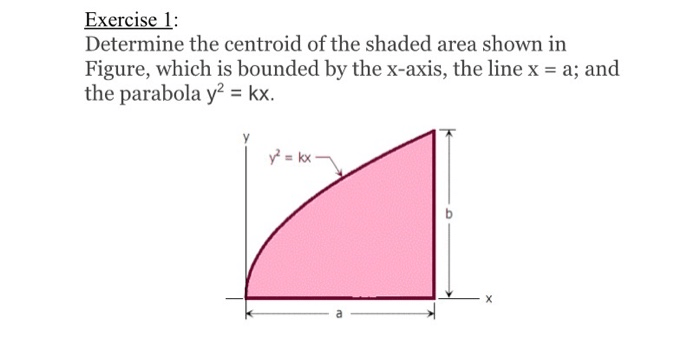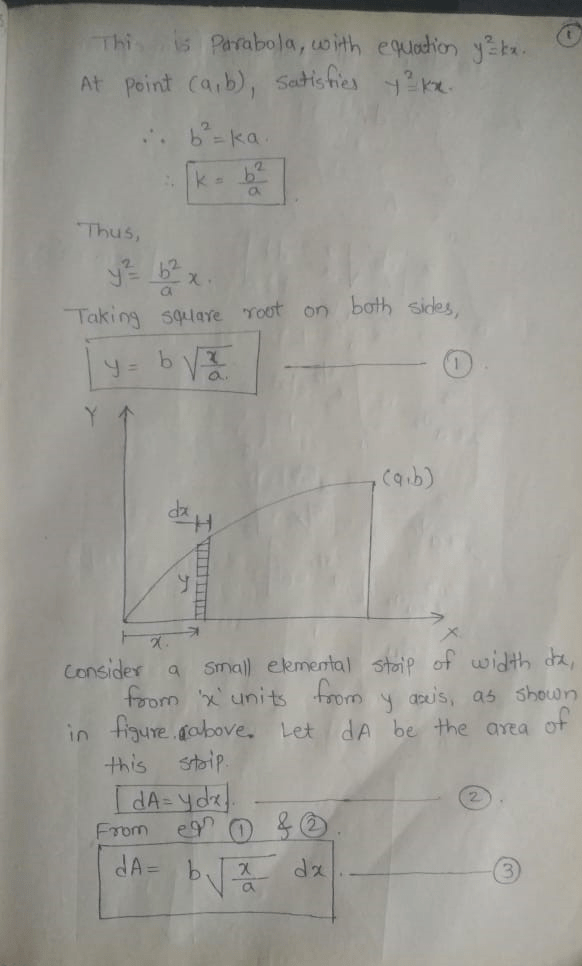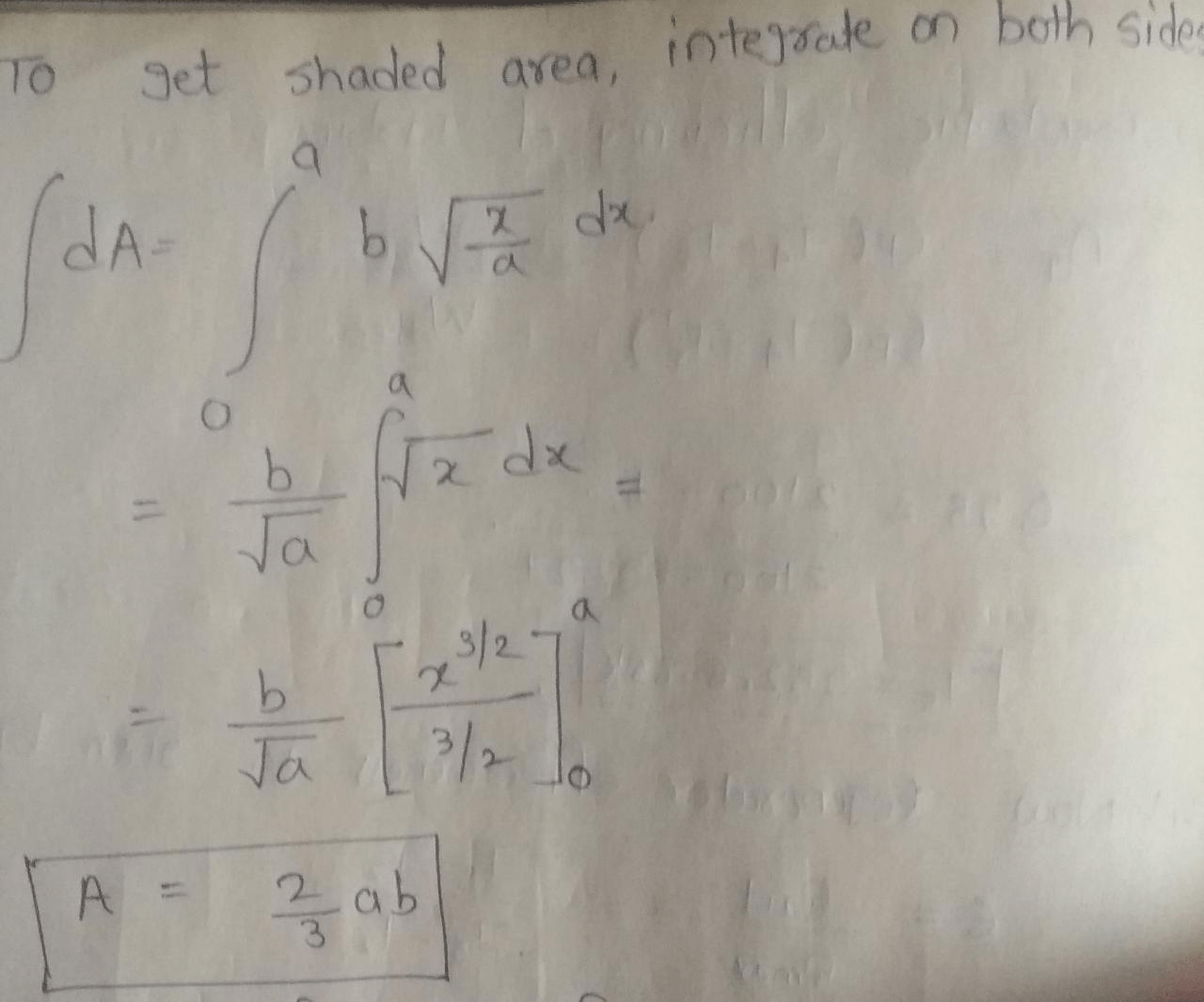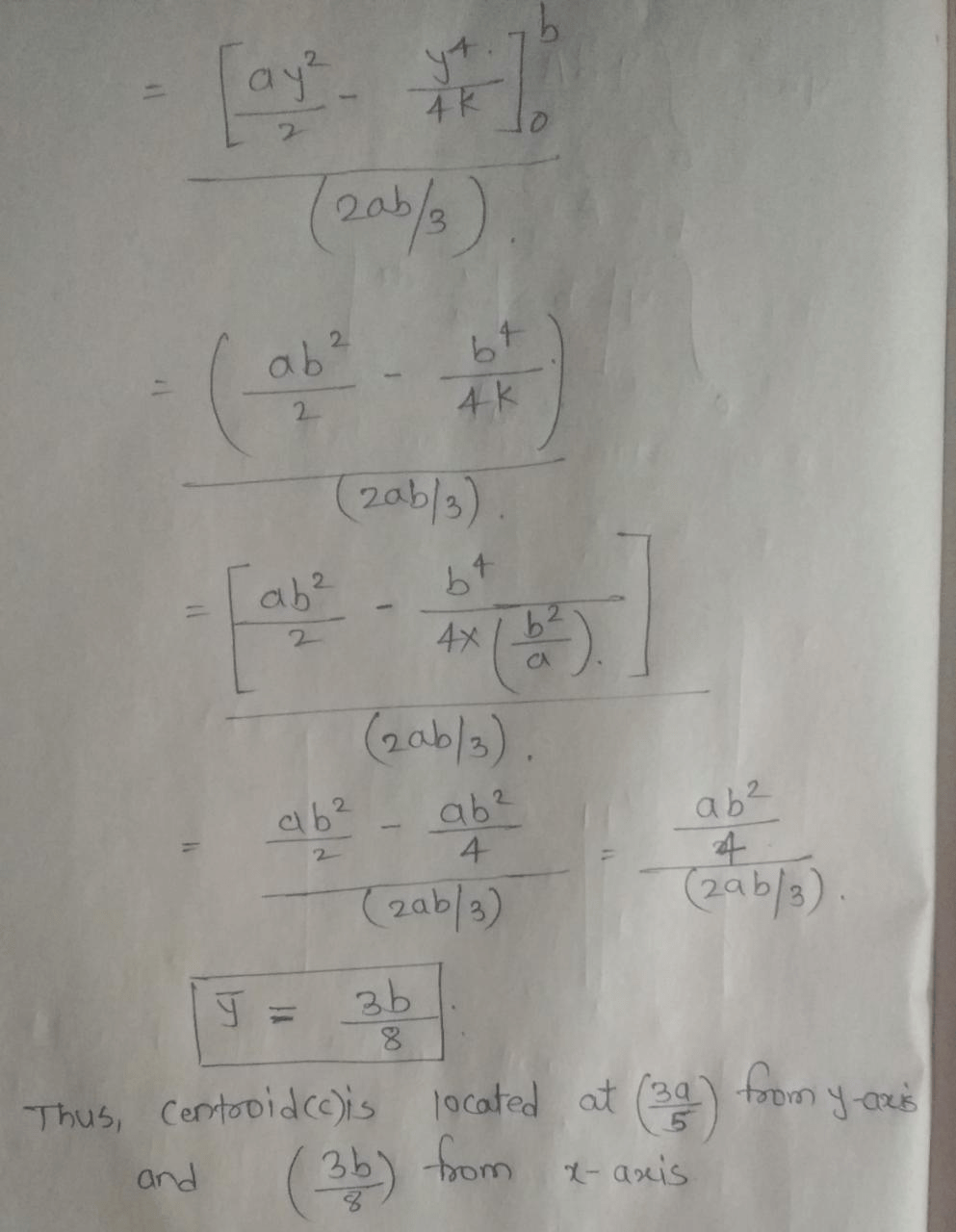# Determine the centroid of the shaded area shown in Figure, which is bounded by the x-axis, the line x = a; and the parabola y2 = kx.

Question-AnswerCategory: Strength of MaterialsDetermine the centroid of the shaded area shown in Figure, which is bounded by the x-axis, the line x = a; and the parabola y2 = kx.

Exercise 1: Determine the centroid of the shaded area shown in Figure, which is bounded by the x-axis, the line x = a; and the parabola y2 = kx.